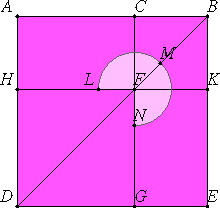# Proposition 4

If a straight line is cut in extreme and mean ratio, then the sum of the squares on the whole and on the lesser segment is triple the square on the greater segment.

Let AB be a straight line cut in extreme and mean ratio at C, and let AC be the greater segment.

I say that the sum of the squares on AB and BC is triple the square on CA.I.46

Describe the square ADEB on AB, and draw the figure.

Since, then, AB is cut in extreme and mean ratio at C, and AC is the greater segment, therefore the rectangle AB by BC equals the square on AC.

And AK is the rectangle AB by BC, and HG is the square on AC, therefore AK equals HG.

And, since AF equals FE, add CK to each, therefore the whole AK equals the whole CE. Therefore the sum of AK and CE is double AK.

But the sum of AK and CE is the sum of the gnomon LMN and the square CK, therefore the sum of the gnomon LMN and the square CK is double AK.

But, further, AK was also proved equal to HG, therefore the sum of the gnomon LMN and the squares CK and HG is triple the square HG.

And the sum of the gnomon LMN and the squares CK and HG is the sum of the whole square AE and CK, which are the squares on AB and BC, while HG is the square on AC.

Therefore the sum of the squares on AB and BC is triple the square on AC.

Therefore, if a straight line is cut in extreme and mean ratio, then the sum of the squares on the whole and on the lesser segment is triple the square on the greater segment.

Q.E.D.

## Guide

#### Use of this proposition

This and the next three propositions are all preparatory to the construction of a dodecahedron in proposition XIII.17.